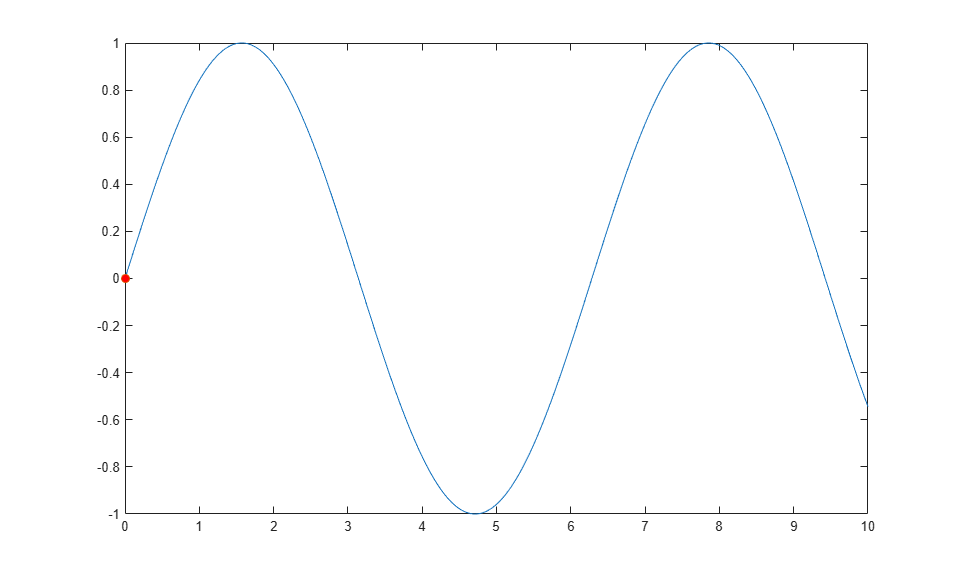# 沿线条跟踪标记

```x = linspace(0,10,1000); y = sin(x); plot(x,y) hold on p = plot(x(1),y(1),'o','MarkerFaceColor','red'); hold off axis manual ``````for k = 2:length(x) p.XData = x(k); p.YData = y(k); drawnow end ```